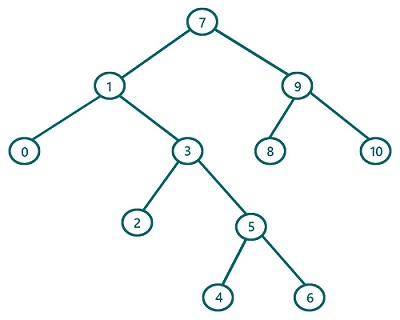# Inorder Traversal in Binary Tree Iteratively (without recursion)

In this article, we are going to find what inorder traversal of a Binary Tree is and how to implement inorder traversal iteratively without using recursion? We have provided the implementation in C++.
Submitted by Radib Kar, on July 30, 2020

In the earlier article on inorder traversal, we saw that inorder traversal is one of traversal which is based on depth-first search traversal.  The basic concept for inorder traversal lies behind its name. "In" means between and that’s why the root is traversed in between its left & right subtree. The basic rule is:

1. First, traverse the left subtree
2. Then traverse the root
3. Finally, traverse the right subtree

Of course, while traversing the subtrees we will follow the same order

We already saw a recursion based implementation. But here, we are going to discuss how to implement without recursion using stack iteratively.When we are using recursion, what we are doing is traversing the left subtree always and then the current root and finally the right subtree. And we do this recursively for the subtrees. Like to traverse the right subtree, we again first traverse its left subtree, then the root and then again its right subtree if there any. Now to do this without recursion, we need stack which will do what recursion does. So the algorithm is:

```1)  Define a stack st first & set the current node as root.
2)  While (current is not NULL or stack is not empty)
//Going as much left as possible always
While(current is not NULL)
Push current to stack st
Current=current->left
End While
Pop stack and print //traversal of nodes
where left subtrees will be traversed first
Set current=current->right //traversing right subtree
End While
```

Dry Run using the above algorithm

Let's dry run the above example, using the above algorithm

We will use the value itself to represent the root

```1)  Define stack st & current is the root(7)
2)  Processing while loop:
---------------------------------------------
Iteration 1(outer loop)

Current is not NULL
Now to process the inner loop:
Current is not NULL
It will push 7 to stack
Then, current=current->left=1

Current is not NULL
It will push 1 to stack
Then, current=current->left=0

Current is not NULL
It will push 0 to stack
Then, current=current->left=NULL
Current is NULL, so inner loop breaks

Now at this point, our stack is,
0 (top)
1
7

So pop from the stack and set current to that node
So current is 0 and traverse/print it //Printed 0
Now, current=current->right=NULL
---------------------------------------------

Iteration 2:
Current is NULL but the stack is not empty
Since Current is NULL thus it will not
fall into the inner loop
Now at this point, our stack is,
1(top)
7

So pop from the stack and set current to that node
So Current is now 1 and traverse (print) it //printed 1
Now, current=current->right=1->right=subtree rooted with 3
---------------------------------------------

Iteration 3:
Current is not NULL
Since Current is not NULL thus it will
fall into the inner loop
Now to process the inner loop:
Current is not NULL
It will push 3 to stack
Then, current=current->left=2
Current is not NULL
It will push 2 to stack
Then, current=current->left=NULL
Current is NULL, so inner loop breaks

Now at this point, our stack is,
2 (top)
3
7

So pop from the stack and set current to the popped node
So current is 2 and traverse/print it //Printed 2
Now, current=current->right=NULL

In this way, iterations will keep happening and will stop
only when both conditions satisfy which are stack is empty
and current is NULL.
It indicates the end of traversal. I would suggest doing the
dry run in paper and pen keeping the stack in front at every
iteration.

Finally we will get traversal as:
0 1 2 3 4 5 6 7 8 9 10

```

C++ implementation:

```#include <bits/stdc++.h>
using namespace std;

// tree node is defined
class TreeNode {
public:
int val;
TreeNode* left;
TreeNode* right;
TreeNode(int data)
{
val = data;
left = NULL;
right = NULL;
}
};

void inorder(TreeNode* root)
{
//base case
if (root == NULL)
return;

stack<TreeNode*> st;
TreeNode* cur = root;
while (cur || !st.empty()) {
while (cur) {
st.push(cur);
cur = cur->left;
}
cur = st.top();
st.pop();
cout << cur->val << " ";
cur = cur->right;
}
}

int main()
{
//building the tree
TreeNode* t = new TreeNode(7);

t->left = new TreeNode(1);
t->left->left = new TreeNode(0);
t->left->right = new TreeNode(3);
t->left->right->left = new TreeNode(2);
t->left->right->right = new TreeNode(5);
t->left->right->right->left = new TreeNode(4);
t->left->right->right->right = new TreeNode(6);
t->right = new TreeNode(9);
t->right->left = new TreeNode(8);
t->right->right = new TreeNode(10);

printf("Inorder traversal of the above tree is:\n");
inorder(t);

return 0;
}
```

Output:

```Inorder traversal of the above tree is:
0 1 2 3 4 5 6 7 8 9 10
```

What's New (MCQs)

Top Interview Coding Problems/Challenges!

Languages: » C » C++ » C++ STL » Java » Data Structure » C#.Net » Android » Kotlin » SQL
Web Technologies: » PHP » Python » JavaScript » CSS » Ajax » Node.js » Web programming/HTML
Solved programs: » C » C++ » DS » Java » C#
Aptitude que. & ans.: » C » C++ » Java » DBMS
Interview que. & ans.: » C » Embedded C » Java » SEO » HR
CS Subjects: » CS Basics » O.S. » Networks » DBMS » Embedded Systems » Cloud Computing
» Machine learning » CS Organizations » Linux » DOS
More: » Articles » Puzzles » News/Updates

© https://www.includehelp.com some rights reserved.